# Consider the following vector ﬁeld. F = (xi + yj + zk )/((x^2 + y^2 + z^2)^3/2) (a) Find the divergence of F. (b) Let S...

Consider the following vector ﬁeld. F = (xi + yj + zk )/((x^2 + y^2 + z^2)^3/2)

(a) Find the divergence of F.

(b) Let S be any sphere not containing the origin. Find the outward ﬂux of F across S.

(c) Let Sa be the sphere of radius a centered at the origin. Find the outward ﬂux of F across Sa.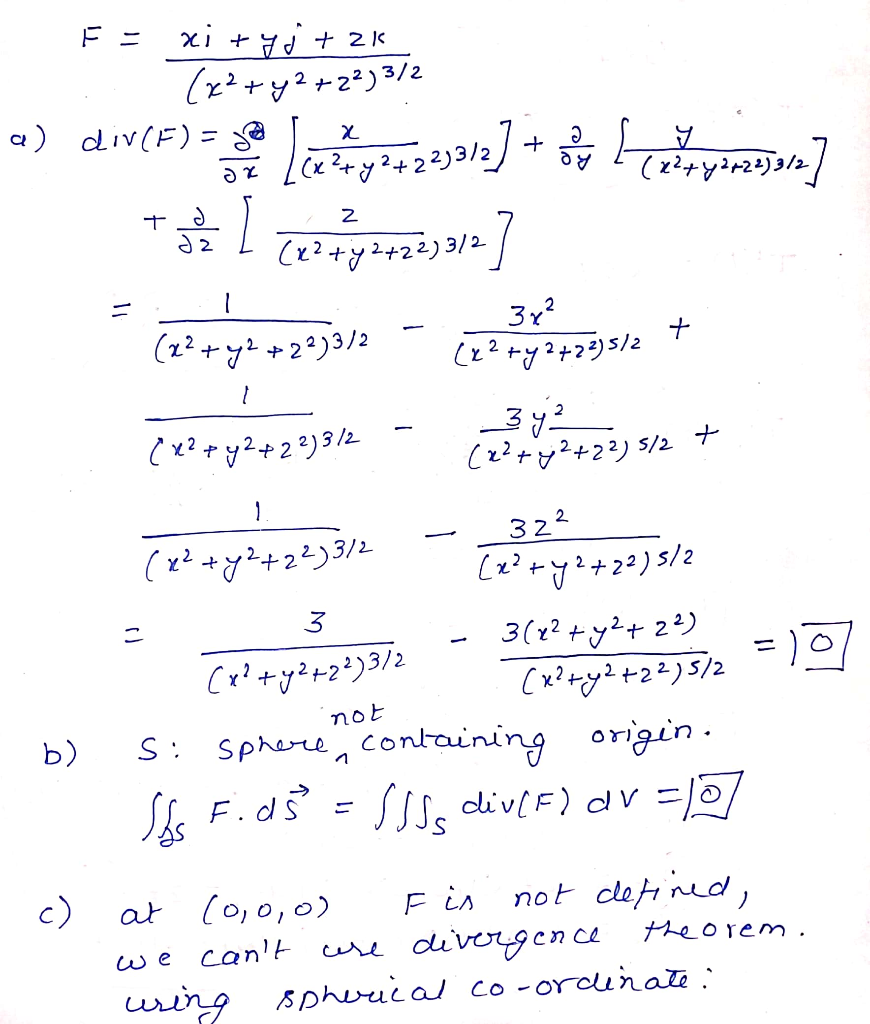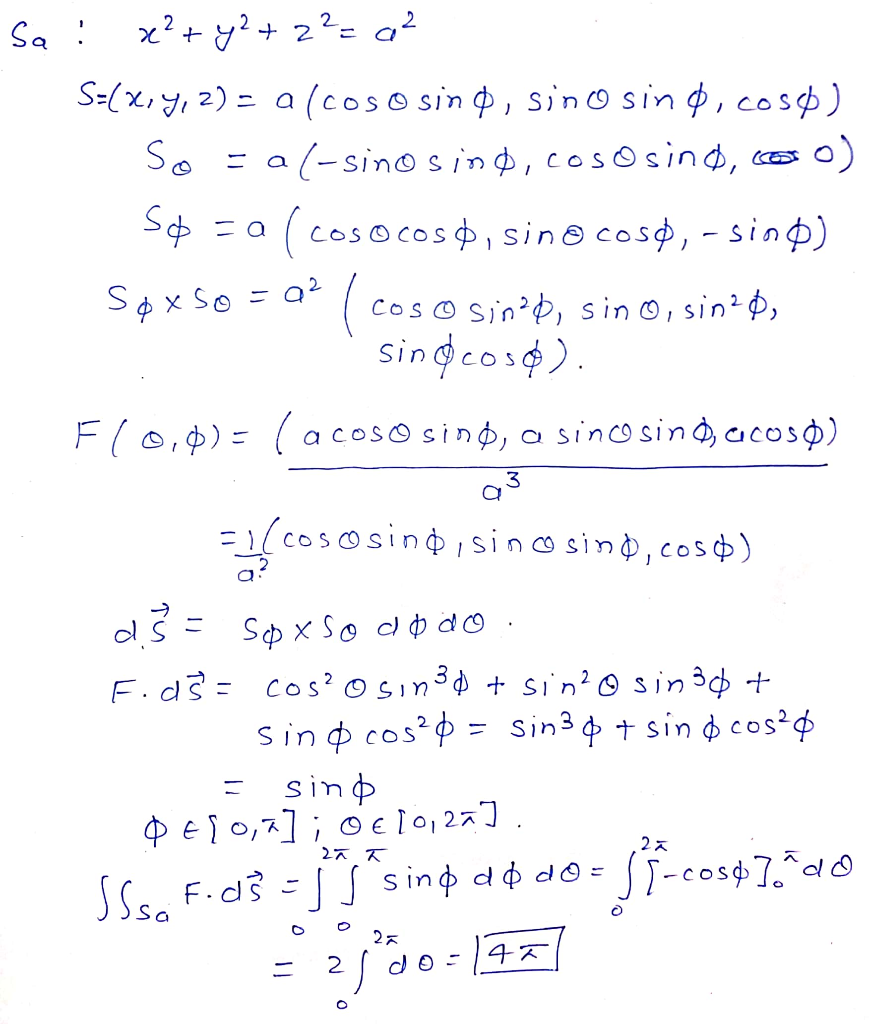##### Add Answer of: Consider the following vector ﬁeld. F = (xi + yj + zk )/((x^2 + y^2 + z^2)^3/2) (a) Find the divergence of F. (b) Let S...
Similar Homework Help Questions
• ### can you solve this vector problems? Find the outward flux of the vector field F(x, y,...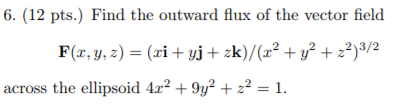can you solve this vector problems? Find the outward flux of the vector field F(x, y, z) = (xi + yj + zk)/(x 2 + y 2 + z 2 ) 3/2 across the ellipsoid 4x^2 + 9y^2 + z^2 = 1. 6. (12 pts.) Find the outward flux of the vector field F(r,y, ) (ri yj+ zk)/(x2 + y2 22)3/2 across the ellipsoid 4r2 +9y2 + z2 = 1 6. (12 pts.) Find the outward flux of the vector...

• ### 9. Let Q be the solid bounded by the cylinder x2 + y2 = 1 and the planes z = 0 and z = 1 . Use the Divergence Theorem to calculate | | F . N dS where s is the surface of Q and F(x, y, z) = xi + yj +...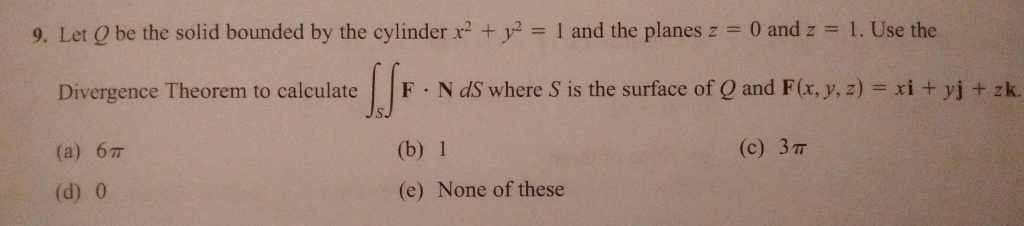9. Let Q be the solid bounded by the cylinder x2 + y2 = 1 and the planes z = 0 and z = 1 . Use the Divergence Theorem to calculate | | F . N dS where s is the surface of Q and F(x, y, z) = xi + yj + zk. (a) 67T (d) 0 (b) 1 (e) None of these (c) 3π 9. Let Q be the solid bounded by the cylinder x2 + y2...

• ### (1) Let G(,y, z) = (x,y, z). Show that there exists no vector field A : R3 -> R3 such that curl(A) Hint: compute its...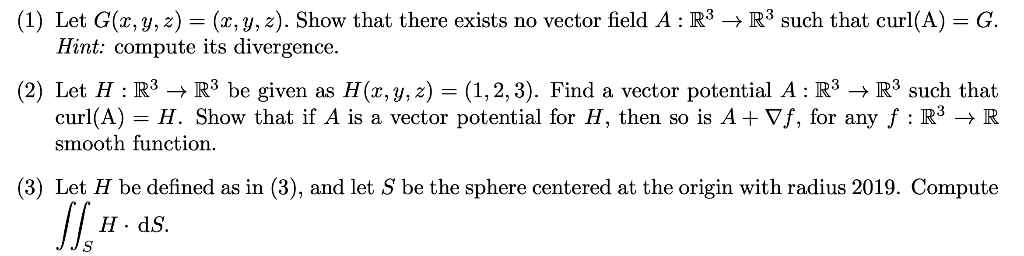(1) Let G(,y, z) = (x,y, z). Show that there exists no vector field A : R3 -> R3 such that curl(A) Hint: compute its divergence G. (2) Let H R3 -> R3 be given as H(x,y, z) = (1,2,3). Find a vector potential A : R3 -> R3 such that curl(A) smooth function = H. Show that if A is a vector potential for H, then so is A+ Vf, for any f : R5 -> R (3) Let...

• ### Let F 9xi + yj + zk . S is the part of the surface z...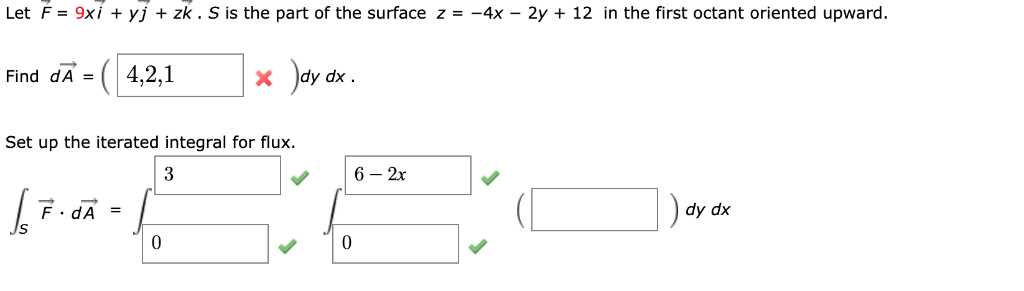Let F 9xi + yj + zk . S is the part of the surface z = -4x - 2y + 12 in the first octant oriented upward. ey 4,2,1 Find dA X * хр / Set up the iterated integral for flux 3 6 2x F.dA dy dx Let F 9xi + yj + zk . S is the part of the surface z = -4x - 2y + 12 in the first octant oriented upward. ey 4,2,1 Find...

• ### Divergence Theorem

F = r/|r|, where r = xi +yj +zk; S is the sphere ρ = 2 of radius 2 centered at the origin.Use the divergence theorem to evaluate the integral of (F dot n) dS, where n is the outer unit normal vector to the surface S.Please explain your steps thoroughly. I don't understand how to use the S parameters to evaluate.

• ### Curl and Divergence of a Vector Field

How do I find the curl and divergence of the vector field F(x,y,z) = {1/√(x2+y2+z2)}*(xi +yj+zk) ?

• ### #4 please 3. (12 pts). (a) (8 pts) Directly compute the flux Ф of the vector field F-(x + y)1+ yj + zk over the closed surface S given by z 36-x2-y2 and z - 0. Keep in mind that N is the outward...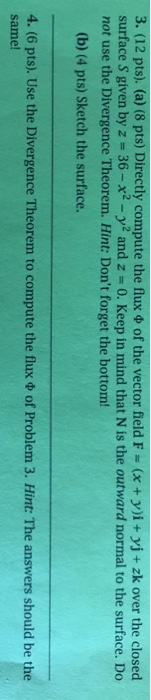#4 please 3. (12 pts). (a) (8 pts) Directly compute the flux Ф of the vector field F-(x + y)1+ yj + zk over the closed surface S given by z 36-x2-y2 and z - 0. Keep in mind that N is the outward normal to the surface. Do not use the Divergence Theorem. Hint: Don't forget the bottom! (b) (4 pts) Sketch the surface. ts). Use the Divergence Theorem to compute the flux Ф of Problem 3. Hint: The...

• ### S: y = 0, y = a, z x=0, x = a, 0.2 = a 14. F(x, y, z) = 4xzi + yjt 4xyk 2 15. F(x, y, z) = z21 + yj + zk S: y...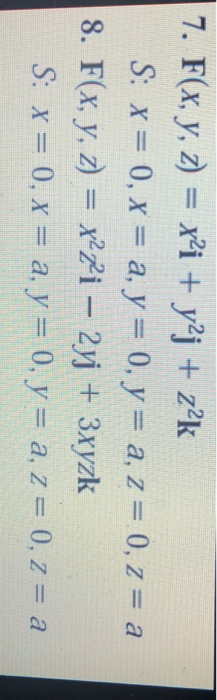S: y = 0, y = a, z x=0, x = a, 0.2 = a 14. F(x, y, z) = 4xzi + yjt 4xyk 2 15. F(x, y, z) = z21 + yj + zk S: y = 0, y = a, z x=0, x = a, 0.2 = a 14. F(x, y, z) = 4xzi + yjt 4xyk 2 15. F(x, y, z) = z21 + yj + zk

• ### Find the outward flux of F(x,y,z)

Find the outward flux of F(x,y,z)=(x+y+z)^(-3/2)*(xi+yj+zk) through the ellipse 4x^2+9y^2+z^2=36Thanks a lot ! I am really confused with it.

• ### (1 point) Compute the flux of F xi + yj + zk over the quarter cylinder S given by x2 + y2 -1, 0 3x s 1,0 <y<1,0 3z< 1, oriented outward flux = (1 point) Compute the flux of F xi + y...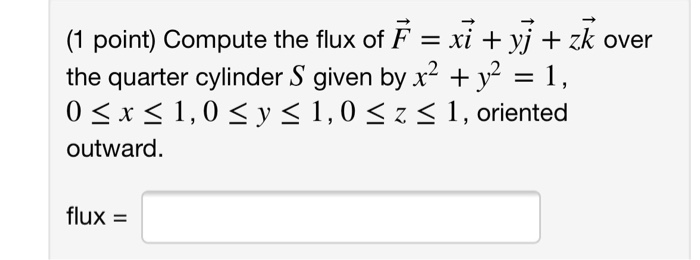(1 point) Compute the flux of F xi + yj + zk over the quarter cylinder S given by x2 + y2 -1, 0 3x s 1,0 <y<1,0 3z< 1, oriented outward flux = (1 point) Compute the flux of F xi + yj + zk over the quarter cylinder S given by x2 + y2 -1, 0 3x s 1,0

Need Online Homework Help?